## MASS TRANSFER

### by Prof. E.R. Laithwaite and W.R.C. Dawson

A SYSTEM FOR THE TRANSFER OF MASS DERIVED FROM
THE PRINCIPLE OF CONSERVATION OF MOMENTUM

The majority of propulsion systems in use today rely either, on exerting forces against the surface over which they travel (e.g. cars, trains, funiculars (via their supporting rope) etc., accelerate material which comprises the medium through which they travel in a direction opposite to the direction in which they are being propelled (e.g. propeller aircraft, power driven or manually propelled boats), take advantage of thermally or gravitationally derived energy gradients (e.g. sailing boats and gliders or surf boards) or eject material in the form of fuel being contained within the vehicle in part as in the case of a jet engine or totally in the case of a rocket engine.) If the vehicle is in space where there is no significant medium with which to react there has been no alternative but to employ the latter method.

When compared to the performance obtainable with a jet engine where a large proportion of the material being ejected from the vehicle is not carried on board but gathered from the atmosphere ahead of the vehicle, the rocket engine is highly inefficient. Energy not directly contributing to the propulsion of the vehicle is lost in heat and light while the huge quantity of volatile fuel required to be carried represents a danger both to the craft and contents. The range and indeed manoeuvrability of the vehicle is totally limited by the amount of fuel carried and indeed once accelerated, deceleration can only be achieved by the expenditure of further fuel.

The work we have been doing at the University of Sussex with gyroscopes, or, in the general sense, flywheels, enables mass to be moved linearly without reaction with the surrounding medium, or ether, and without projection or loss of mass from the total. Where there is an existing medium, i.e. on land, sea or air, the rate of generation of momentum appears to be unexciting. However, in the absence of a medium, in space, out of easy reach and requiring extended ability to manoeuvre, this system does not merely come into its own, it is unique. For the first time, it offers a means of imparting linear movement by the delivery of small "parcels" of instantaneous momentum to a vehicle in a manner not too dissimilar from the current way in which space vehicles are re-oriented by the application of the principle of the conservation of angular momentum. Now we can offer a device that will enable a vehicle metaphorically to "Swim through Space".

EXPLANATORY INTRODUCTION TO MASS DISPLACEMENT

MOVING AN OBJECT WITHOUT PUSHING IT.

When we wish to move an object that appears to be stationary with respect to us, it is our common experience that it is necessary to apply a force to cause it to do so. This is because the object possesses mass which gives to the object that characteristic of resisting having its velocity changed, known as inertia. The relationship between these properties was discerned by Newton and is usually written (Force) = (Mass) x (Acceleration).

What Newton actually stated was:- "The force is proportional to the rate of change of Momentum"

So what is momentum, why bother with it?

The Ultimate law concerning energy is that it can neither be created or destroyed. It has to come from somewhere and must be absorbed somehow. It is never just 'lost'. Surely this criterion alone should enable us to solve all our problems in mechanics?Consider the problem of a bullet being fired into a stationary block of wood, as in figure 1.

At a), a bullet of mass m is fired with velocity v into a block of mass M

At b), the bullet is embedded in the wood and both are travelling at a combined velocity V.

How do we calculate V?

On the face of it, we only need to equate the kinetic energy before and after the impact, since energy cannot be destroyed and solve for V.

And when we come to measure V practically, we find it is less than that predicted by this approach. . . . WHY? The answer is that some of the kinetic energy of the bullet was used in embedding itself into the wooden block and in creating heat and sound as it did so. How much of the energy was converted - we cannot tell!

Newton spotted this difficulty. Energy has many forms and can be converted from one to another. It exists as a potential; as heat, light, sound, electrical and magnetic as well as kinetic energy. Newton said in effect 'if instead of conserving energy you conserve momentum, you will get the right answer, for momentum is not convertible to any other form.

Now, by equating momentum before and after the impact,

mv = (M + m)V
and solving for V, we get the right answer.

STARTING AND STOPPING.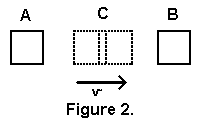Referring to figure 2, the objective is always to move from A to B, starting at rest and finishing at rest. If A and B are at the same potential there has been no change in energy so, theoretically, no energy should be required to make the journey. Yet, in order to move, you have to attain a velocity at C. So, from A to C there must have been an acceleration. This needed a force to produce it. Providing a force on moving objects requires work, that is an energy input.

Suppose the object of mass m attains its maximum speed v at the centre of the journey C. The work done has appeared as the kinetic energy (mv^2)/2. Now it has to be slowed so as to come to rest at B. This also required a force, - a reverse force. - apparently requiring more energy; but in theory this kinetic energy could be recaptured.Consider for example, that the initial acceleration could be provided by a spring, as in figure 3. The energy stored in the spring as potential energy is converted into kinetic energy represented by (mv^2)/2. At B it will again come to rest having compressed the second spring an equal amount and converted all the kinetic energy back into potential energy.

If the anchor point of the spring could now be moved by rotation of 180 degrees to C', it could be used to drag the mass still further to the right and so on. This cannot be used as a space drive because the spring has to have an anchor point to act against; in space there is no anchor point nor is there a means of moving it if there had been.

ACTION AND REACTION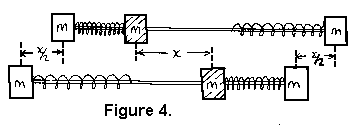Figure 4. shows two blocks each of mass m joined by a light rod. Another mass, also m, can slide along the rod. A spring us used to propel the sliding mass from A to B. At B the sliding mass will have compressed the second spring to recover its kinetic energy. How will the whole combination have moved?

Let the accelerating force being applied by the spring be F. The initial acceleration of the sliding mass is F/m. The two joined masses accelerate in the opposite direction at F/2m. At the end of the acceleration, i.e. at the mid point C, the sliding mass has moved a distance x in one direction while the joined masses have moved a distance x/2 in the opposite direction.

You can now see that the centre of mass has not moved at all. m moved a distance x in one direction and 2m moved a distance x/2 in the opposite direction.

The same is true for the stopping part of the journey. Apparently we are therefore totally incapable of projecting anything which is totally enclosed in a box. Only by projecting material out of the box into space (like a rocket) can we move the box.

LINEAR MOTION AND CIRCULAR MOTION

Linear motion involved quantities such as:-

Linear Displacement (Distance)

Linear Velocity (Rate of Change of Distance with Time)

Linear Acceleration (Rate of Change of Velocity with Time)

Linear Force (Mass times Acceleration)

Linear Momentum (Mass times Velocity

Circular motion has corresponding quantities:-

Angular Displacement (Angle)

Angular Velocity (Rate of Change of Angle with Time)

Angular Acceleration (Rate of Change of Angular Velocity with Time)

Angular Force (Torque)

Angular Momentum (Angular Velocity times (Angular Mass), or Moment of Inertia)

In conserving momentum, apparently, we are constrained to conserve linear and angular momentum separately. If a spinning object moves in a straight line the momentum of spin contributes nothing to the linear momentum and vice versa.Similarly, if a person stands on turntable T, as shown in figure 5, and rotates once per second, whether the turntable stays where it is or whether it moves at any velocity, the person on the turntable will still face the wall W once every second.

AND YET....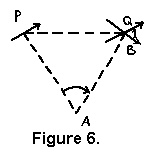If an object is moved in a straight line, as in figure 5, this essentially linear motion can be achieved by two pure rotations. In figure 6, an object is being moved from P to Q and this is achieved by two rotations, one about A and the other about B.

MASS DISPLACEMENT BY CIRCULAR MOTION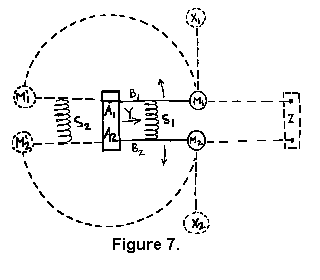So, is it possible to circular motion to obtain mass displacement? An arrangement such as in figure 7. looks promising. The two masses M1 and M2 are initially propelled sideways, as by a compressed spring S1. Thereafter, they rotate about centres A1 and A2, constrained only by the two light rods B1 and B2, arriving at M1' and M2' where their kinetic energy is recovered in a second spring S2. Have the masses moved across space from M1, M2 to M1', M2'?

Actually no! All the time the masses are rotating about the centres A1 and A2 they are exerting centrifugal forces on a1 and a2. For the first 90 degrees of rotation they accelerate the centre pivot anchor block containing A1 and A2 in the direction of arrow Y. For the second 90 degrees of rotation they decelerate the centre pivot anchor block to rest. All that happens (provided the mass of the pivot block is zero) is that the masses M1 and M2 will move out sideways to positions X1 and X2 and then return to their original positions. The pivot block meanwhile, moves from its original position Y to position Z, attaining a maximum velocity as it passes between the two masses which are then at X1 and X2.

No mass transfer takes place. Even if the block itself had mass the centre of mass of the whole system would not move.

THE BREAKOUTWe discovered that, if the masses M1 and M2 were not 'dead' masses but were spinning wheels, (shown edge on in figure 8), the pivot block would not be displaced from Y to Z, as in figure 7, but some smaller distance Z1. Thus, even if the pivot block has mass, the result of 180 degrees rotation of the masses M1 and M2 about A1 and A2 is that the mass centre has been moved to the left.

Experiments indeed show that the centrifugal forces demanded by spinning wheels precessed in this manner are less than the values calculated from simple mechanics. The reduction in centrifugal force depends on the "quality" of the wheels and the manner of precession. The quality of the wheel depends on the relative size of the rim to its diameter and the proportion of its mass that is in that rim. Thus, the finer the rim and the more of its mass is in the rim, the "better" the wheel will be. Likewise, the faster the rate of spin is to the rate of precession, and the larger the radius of the spinning wheel is to the radius of precession, the less will be the centrifugal force it exhibits.

To get an impressive reduction in centrifugal force you need a large heavy wheel with a very thin rim, virtually no spokes or middle, running very fast and being precessed slowly round a small radius.

Experimentally, with a high quality wheel, precessing on a radius about twice that of the wheel, with a spin in excess of 100 times the rate of precession, we have recorded centrifugal forces of less than one tenth of that anticipated from calculation.

EXPLOITATION

Mass displacement is effected without force on the framework. Spinning wheels do not deal in linear quantities, including linear force. In returning the mass to its initial spatial position, any means can be employed that does not deflect the axis of spin. Such return motion produces reaction force, even against a resisting force. If a resisting force is present, the work done against that resistance is supplied by the mechanism, not the wheel. At no time during the recovery journey does the device as a whole have any linear momentum.

If the motion of the precessional and conventional linear displacement are combined in a cyclical manner - and there are many way of doing this - it is possible to produce a continuous sinusoidal displacement and therefore, in a polyphase version, continuous steady displacement. The moment the mechanism stops, so does the motion. The mechanism is no more capable of producing kinetic energy than it is of producing momentum.

BUT, repositioning of mass, even against resisting forces, is positively attainable.

TORQUE AND PRECESSION

Experiments with inertial masses performed in a terrestrial laboratory are liable to be dominated by gravitational forces which are large in comparison with those being measured.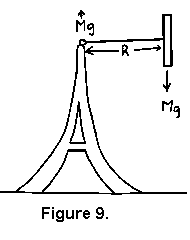Toy gyroscopes, made to precess round models of the Eiffel Tower, do so as a result of the torque MgR (see figure 9) derived from the mass of the gyroscope, and the thrust upward exerted by the support on the tower. Experience with gyroscopes of this type gives rise to the idea that torque is the cause of a precession.

A similar idea persists in electrical engineering when a voltage is seen to the cause of electric current. This is the result of our commercial and household electrical devices all being connected in parallel to a source of voltage where the current drawn by individual items depends on the resistance, so that I = e/R.

Had we elected to send a fixed current through all our devices in series, the voltage developed across an individual item would be given by e = iR and would be seen as the result of the current rather than its cause.

In the same way, a gyroscope can be forced to precess in a predetermined path. If the torque so generated is resisted by the framework (or even another gyroscope) and is measured, it will be found that the gyroscope will have demanded just the torque required to make it so precess and that the precession is, in this case the cause of the torque.

LOCATION OF AXIS

A gyroscope, which is really only a flywheel, is essentially a 3-dimensional device. Just as the direction of rotation of an electric motor can be determined from the directions of magnetic field and the electric current, by holding two fingers and the thumb mutually at right angles in the well-known 'Fleming's Left Hand Rule', so the directions of spin, momentum and torque may be used to predict the direction of precession.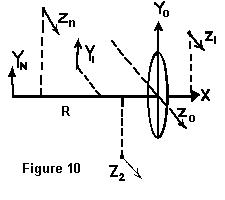If the spin momentum of the wheel is in direction X, (see figure 10), then the torque applied about an axis in Y, at right angles to the plane of the drawing, will produce precession about an axis such as Z, perpendicular to the plane of the drawing.

Interestingly, the gyroscope appears to be unconcerned about the precise location of the axis in Y, which may be at Y1 or Y2 or etc. . . Yn, so long as they are parallel. Likewise the resulting precession can take place about Z, or Z1 or Z2 or etc. . . Zn. No particular axis in Y is related to any particular axis of Z.

The following equation is universal.

Torque (about any axis in the Y plane) = Angular-Momentum (of the gyro) x Precessional Angular-Velocity (about any axis in the Z plane)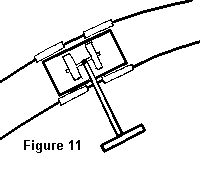Thus a toy train on a continuously curved track, as shown in figure 11, will be propelled around the track and even uphill, by an arm R carrying a spinning wheel W, which is pivoted so as to be free to fall vertically under gravity but transmits its torque horizontally to the truck. Within limits, this is independent of the radius of the track. It does not even require R to be exactly at right angles to the tangent to the track, as was first demonstrated to us in 1975.

It is precisely this property that allows mass displacement to take place, as in figure 6, as the result of two simple rotations about parallel axes through A and B. the essential difference between precession about an axis Yo, that passes through the centre of mass of the spinning wheel, and precession about any other axis Yn is that the wheel has to be given the appropriate amount of linear momentum Mv, in the Z direction such that this tangential precessional velocity v shall be equal to the distance R times the angular velocity. It is this linear momentum that is carried by the angular momentum and which enables mass displacement to be achieved.

E.R. Laithwaite
W.R.C. Dawson
September 1994

Pictures reduced in size, touched up, and coded into this document
by David E. Cowlishaw, 24 March 1998, for inertial propulsion researchers.
Gyroscopic Inertial Thruster Page